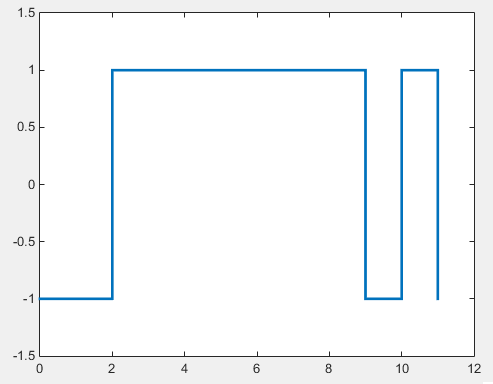Octave/Matlab - Plot Application                                          Home : www.sharetechnote.com

In this page, I would post a quick reference for Matlab and Octave. (Octave is a GNU program which is designed to provide a free tool that work like Matlab. I don't think it has 100% compatability between Octave and Matlab, but I noticed that most of basic commands are compatible. I would try to list those commands that can work both with Matlab and Octave). All the sample code listed here, I tried with Octave, not with Matlab.

There are huge number of functions which has not been explained here, but I would try to list those functions which are most commonly used in most of matlab sample script you can get. My purpose is to provide you the set of basic commands with examples so that you can at least read the most of sample script you can get from here and there (e.g, internet) without overwhelming you. If you get familiar with these minimal set of functionality, you would get some 'feeling' about the tool and then you would make sense out of the official document from Mathworks or GNU Octave which explains all the functions but not so many examples.

I haven't completed 'what I think is the minimum set' yet and I hope I can complete within a couple of weeks. Stay tuned !!!

Plot Applications

<Vector Plot - Vector Field >

Ex)

 Input v = -1:0.2:1; [x,y] = meshgrid(v); dx=y;dy=-x - 0.25 .* y; quiver(x,y,dx,dy); axis([-1 1 -1 1]); OutputEx)

 Input [X,Y] = meshgrid(-2:.2:2); Z = X.*exp(-X.^2 - Y.^2); [DX,DY] = gradient(Z,.2,.2);   subplot(1,2,1); surface(X,Y,Z); view(-20,10);   subplot(1,2,2); contour(X,Y,Z); hold on quiver(X,Y,DX,DY); colormap hsv hold off Output< Vector Plot - Surface Normal >

Ex)

 Input [X,Y] = meshgrid(-2:0.25:2,-1:0.2:1); Z = X.* exp(-X.^2 - Y.^2); [U,V,W] = surfnorm(X,Y,Z); quiver3(X,Y,Z,U,V,W,0.4); hold on surf(X,Y,Z); colormap hsv view(-35,45) axis ([-2 2 -1 1 -.6 .6]) hold off Output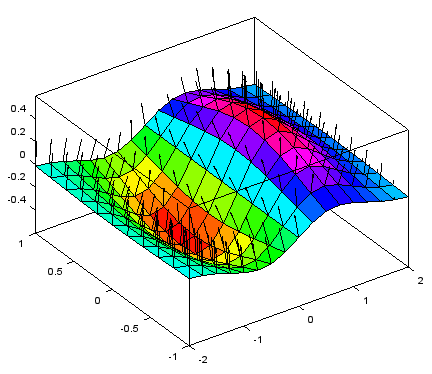< Histogram - Un normalized >

Ex)

 Input N=1000000;   variance = 0.5;   x = sqrt(variance)*randn(1, N);   step = 0.05; range_n = -1.6:step:1.6; range_r = 0:step:3;   hx = hist(x,range_n);   plot(range_n,hx,'b-');xlim([-1.5 1.5]); Output< Histogram - Normalized >

Ex)

 Input N=1000000;   variance = 0.5;   x = sqrt(variance)*randn(1, N); hx = hx/(step*sum(hx));   step = 0.05; range_n = -1.6:step:1.6; range_r = 0:step:3;   hx = hist(x,range_n);   plot(range_n,hx,'b-');xlim([-1.5 1.5]); Output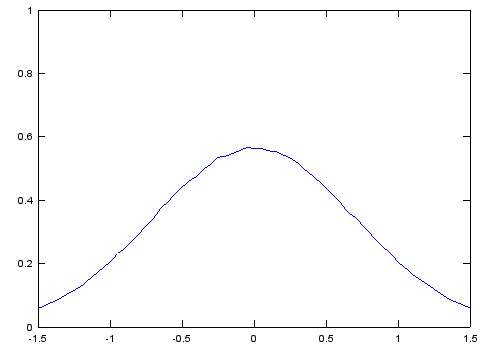< stairs >

Ex)

 Input x = [-1 1 1 -1 1 -1]; stairs(x); ylim([-1.5 1.5]); Output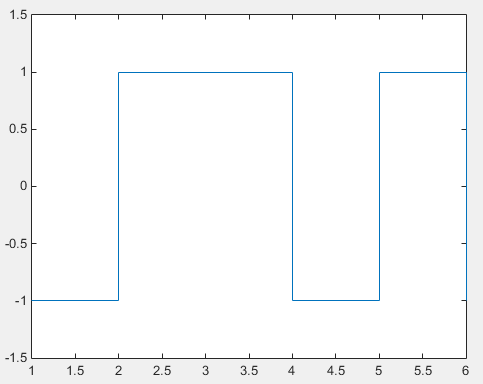Ex)

 Input x = [-1 1 1 -1 1 -1]; stairs(x,'LineWidth',2); ylim([-1.5 1.5]); Output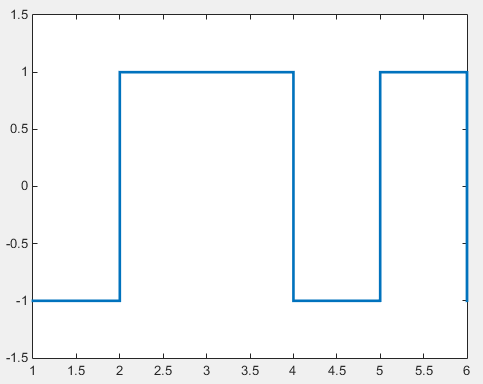Ex)

 Input y= [-1 1 1 -1 1 -1]; x = [0 2 7 9 10 11]; stairs(x,y,'LineWidth',2); ylim([-1.5 1.5]); Output